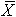# %Off-Target

%Off-Target is a diagnostic measure that represents the percent of the specification range the mean falls from target. This measure is calculated by the following formula.where

• USL is the Upper Specification Limit,
• LSL is the Lower Specification Limit,
• T is the Target, and
•is the sample mean from all combined data.

As the process average departs from target, Ppm is reduced. The figure below graphically displays the relationship between Ppm and %Off-Target. The resulting Ppm is shown for four levels of Pp (at infinity, 3, 2, 1.5, 1) as the offset from target varies. The highest line represents a process with a standard deviation of zero (Pp at infinity). If a process is running with a mean 10% off target, the largest Ppm that may be achieved is 1.67. At 30% off target, the largest Ppm that may be achieved is 0.56.